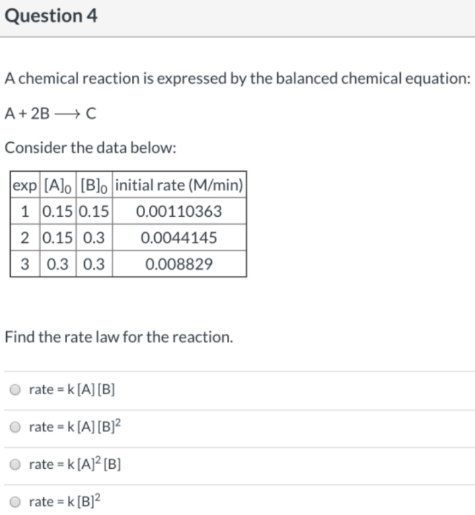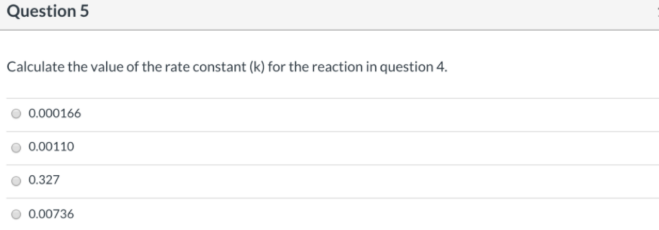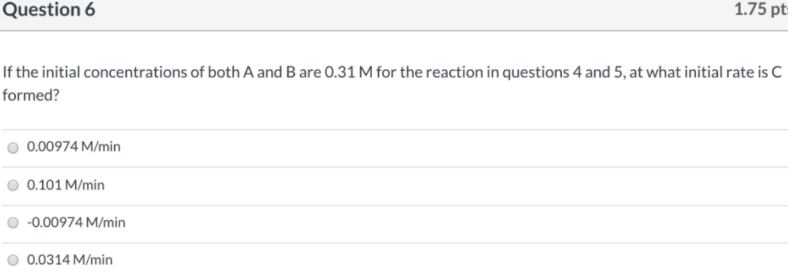# A reaction is expressed by the balanced chemical equation: A + 2B → C Consider the data below: Find the rate law for the reaction. a) rate = k [A] [B] b) rate = k [A] [B]^2 c) rate = k [A]^2 [B] d) rate = k [B]^2 Calculate the value of the rate constant (k) for the reaction in question 4. a) 0.000166 b) 0.00110 c) 0.327 d) 0.00736 If the initial concentrations of both A and B are 0.31 M for the reaction in questions 4 and 5, at what initial rate is C formed? a) 0.00974 M/min b) 0.101 M/min c) -0.00974 M/min d) 0.0314 M/min